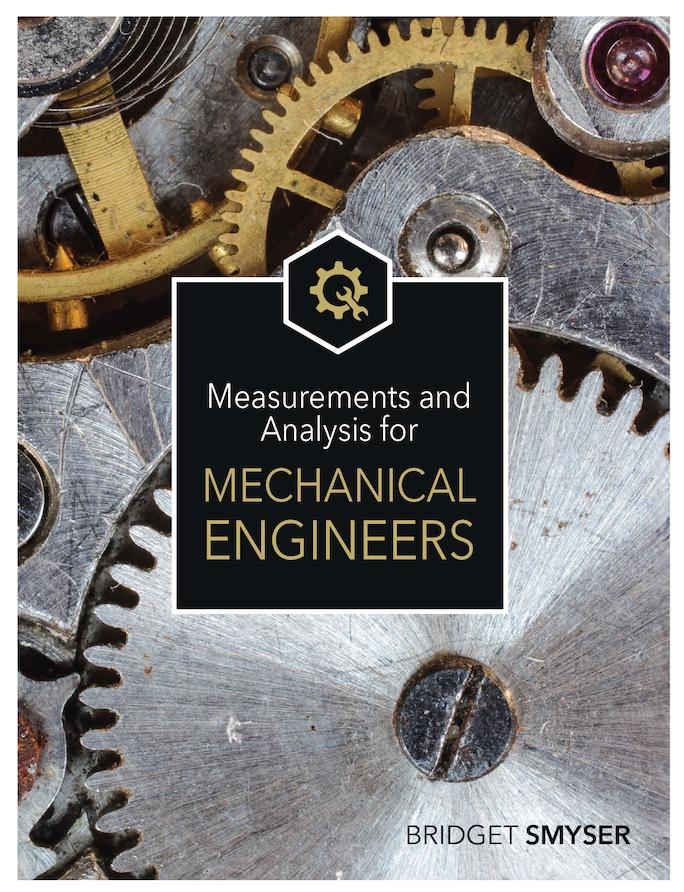# Measurements and Analysis for Mechanical Engineers

An online textbook geared toward mechanical engineers that teaches experimental design, data analysis, uncertainty concepts, and key measurement techniques.

Chapter 1: Introduction to Measurements
How and why we measure
Measurement systems
Variables
Units and Standards
Image sources
References

Chapter 2: Instrument Characteristics
What do we need to know about sensors?
Accuracy and precision
Demystifying specification sheets
Sensitivity and common errors
Types of Instruments
Image sources
References

Chapter 3: Calibration and Standards
Standards
Basic theory of calibration
Calibration methods for common measurements
Calibration for pressure measurement
Calibration for temperture measurement
Calibration for strain, mass, and load measurement
Calibration for pH measurement
Calibration for sound measurement
Calibration for flow rate measurement
Image sources
References

Chapter 4: Design of Experiments
Big picture of Design of Experiments
Parameter Design
Examining the need for the experiment
Defining the objectives for the experiment
Determining what you want to measure
System and Tolerance Design
Identifying the important variables
Designing the experiment
Performing the experiments
Data Reduction Design
Analyzing the results
Acting on the results
Reporting the results
Image sources
References

Chapter 5: Uncertainty
How close can we get?
Design stage uncertainty
Multiple measurement analysis
Error propagation
Reducing and eliminating errors
Summary
Image sources
References

Chapter 6: Statistical Data Analysis
Introduction to statistical measurement theory
Infinte statistics and the normal distribution function
Histograms
Finite statistics
Comparing samples using t-tests
Two-tailed t-tests
One tailed t-tests
t-tests between two samples
Data analysis in Microsoft Excel
Analysis of variance (ANOVA)
Correlation analysis
Testing for normality
Comparing histograms
Normal probability plots
Chi-squared analysis for goodness of fit
Number of data points needed for significant results
Identifying outliers
Summary
Image sources
References

Chapter 7: Dynamic Measurements
General model for measurement system behavior
Zero order systems
First order systems
Step function input
Pulsed input
Ramp input
Wave function input
Second order systems
Step function input
Wave function input
Special case: Using system behavior to study heat transfer
Summary
Image sources
References

Chapter 8: Electrical Measurements and Signal Processing
Basic definitions
Signal characteristics
Periodic signals and Fourier analysis
Sampling theory
Useful circuit concepts
Amplifiers
Filters
Special purpose circuits
Errors in electrical measuremetns
Electrical measurement devices
Image sources
References

Chapter 9: Strain and Related Measurements
Review of stress-strain concepts
Relating strain to electrical resistance
Wheatstone bridge circuits for strain measurements
Quarter bridge circuits
Half bridge circuits
Full bridge circuits
Strain gauge specifications
Practical considerations for strain gauges
Applications of strain gauges
Summary
Image sources
References

Chapter 10: Temperature and Related Measurements
What is temperature?
Errors in temperature measurement
Methods for measuring temperature
Thermal expansion measurements
Thermoelectric measurements
Thermal resistance measurements
Measuring thermal and transport properties
Thermal conductivity
Viscosity
Diffusion coefficients
Summary
Image sources
References

Chapter 11: Pressure and Related Measurements
Pressure scales and units
Types of pressure instruments
Hydrostatic pressure instruments
Aneroid pressure instruments
Static calibration
Dynamic pressure measurements in flowing fluids
Fluid flow over objects
Transmission effects in dynamic pressure measurements
Summary
Image sources
References

Chapter 12: Fluid Flow Measurement
Air flow measurement
Thermal anemometry
Doppler anemometry
Particle image velocimetry
Mass flow measurement
Volumetric flow rate measurement
Differential pressure meters
Turbine flow meters
Rotameters
Drag based meters
Electromagnetic flow meters
Ultrasonic flow meters
Calibration of flow meters
Practical considerations for flow measurements
Image sources
References